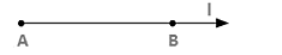Deepak Scored 45->99%ile with Bounce Back Crack Course. You can do it too!

# Define the following terms.

Question:

Define the following terms.

(i)  Line segment

(ii) Collinear points

(iii) Parallel lines

(iv) Intersecting lines

(v) Concurrent lines

(vi) Ray

(vii) Half-line

Solution:

(i) Line-segment:

Give two points A and B on a line I. the connected part (segment) of the line with end points at A and B is called the line segment AB.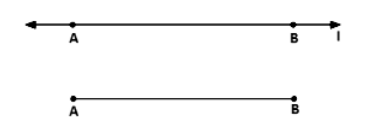(ii) Collinear points:

Three or more points are said to be collinear if there is a line which contains all of them.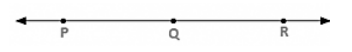(iii) Parallel lines:

Two lines l and m in a plane are said to be parallel lines if they do not intersect each other.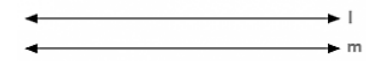(iv) Intersecting lines:

Two lines are intersecting if they have a common point. The common point is called point of intersection.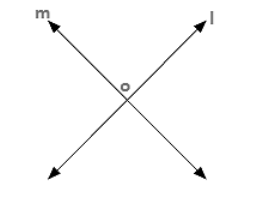(v) Concurrent lines:

Three or more lines are said to be concurrent if there is a point which lies on all of them.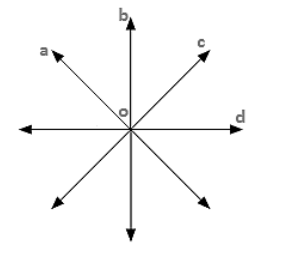(vi) Ray:

A line in which one end point is fixed and the other part can be extended endlessly.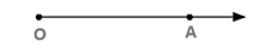(vii) Half-line:

If A, B. C be the points on a line l, such that A lies between B and C, and we delete the point A from line l, the two parts of l that remain are each called half-line.